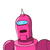# In triangle OPQ, right angled at P, OP-7cm and OQ-PQ = lem determine the value of Sin 8 and Cos 0.​

In triangle OPQ, right angled at P, OP-7cm and OQ-PQ = lem determine the value of Sin 8 and Cos 0.​

### 1 thought on “In triangle OPQ, right angled at P, OP-7cm and OQ-PQ = lem determine the value of Sin 8 and Cos 0.​”

1.Step-by-step explanation:

In Δ OPQ, we have

OQ

2

=OP

2

+PQ

2

⇒(PQ+1)

2

=OP

2

+PQ

2

[∵OQ−PQ=1⇒OQ=1+PQ]

⇒PQ

2

+2PQ+1=OP

2

+PQ

2

⇒2PQ+1=49

⇒PQ=24cm

∴OQ−PQ=1cm

⇒OQ=(PQ+1)cm=25cm

Now, sinQ=

OQ

OP

=

25

7

and, cosQ=

OQ

PQ

=

25

24